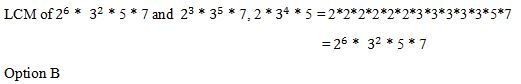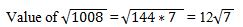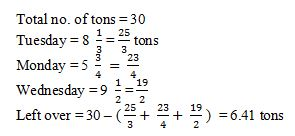# DXC Technology Quantitative Ability Previous Year Questions

by Ajinkya Kulkarni | Updated on 03 October 2023

DXC Technology Quantitative Ability Previous Year Questions

DXC Technology Quantitative Ability Questions

Q1) Arrange the following in ascending order:

2/15, 18/29, 7/18, 10/87.

A. 7/18 < 2/15 < 18/29 < 10/87

B. 10/87 < 7/18 < 2/15 < 18/29

C. 10/87 < 2/15 < 7/18 < 18/29

D. 18/29 < 10/87 < 2/15 < 7/18

Solution : The terms in the required order are 10/87, 2/15, 7/18, 18/29.

To arrange the terms from lowest to greatest, we will have to convert them into like terms.

So, we will find the LCM of the numbers' denominators.

The common and smallest multiple of 15, 29, 18, and 87=2×3×3×5×29

=2610

The terms will change as follows-

2×174/15×174=348/2610

18×90/29×90=1620/2610

7×145/18×145=1015/2610

10×30/87×30=300/2610

We will now compare the numerators of these numbers and arrange them accordingly.

The arrangement becomes: 300/2610, 348/2610, 1015/2610, 1620/2610

= 10/87, 2/15, 7/18, 18/29

Therefore, the terms in the required order are 10/87, 2/15, 7/18, 18/29.

Option C.

Q2) Find the L.C.M. of 26 * 32 * 5 * 7, 23 * 35 * 7 and 2 * 34 * 5.

A. 26 * 35 * 7

B. 26 * 35 * 5 * 7

C. 26 * 35 * 5

D. 26 * 35

Solution:Q3) The value of log8(1/4096) is

A. -5

B. -2

C. -4

D. -3

Solution:

Formula:
logb(x) = y, if by = x

Input:
x = 1/4096 = 8-4
b = 8

Solution:
y = log8 (1/4096)
= log8 8-4
= -4 log8 8 = -4 x 1
log8 4096 = -4

Option C

Q4) If the G.C.D. of x2 + (p - 1) and x2 + px + (p - 1) is x - 1, then the value of p is?

A. 2

B. 3

C. 4

D. 5

Solution:

We all know that the GCD of two polynomials means that it is a common factor of both the polynomials.

Here we are given: GCD = x+1

this means that (x+1) is a factor of both the polynomials.

i.e.  x = -1 is a zero of both the polynomial.

This implies on putting x=-1 in polynomial equation x² - px – 4

we have polynomial equation will be = zero.

x² - px - 4 = 0 at x = -1

1 - (-1)p - 4 = 0

1 + p - 4 = 0

p = 3

similarly, on putting x = -1 in the second polynomial and equating it equal to zero, we will have p = 3

Q5) A bag contains coins of Re. 1, Rs. 2 and Rs. 5 in the ratio of 4:8:5. The total amount in the bag is Rs. 90. The total number of Rs. 5 coins is

A. 15

B. 10

C. 8

D. 16

Solution :

The given ratio is 4 : 8 : 5

Let the number of 1 rupee coin is 4x, number of 2-rupee coin is 8x and number of 5-rupee coin is 5x

Number of coins = 4x + 8x + 5x = 17x

Total amount = 1 * 4x + 2*8x + 5*5x = 90 (given)

45x = 90

X = 2

So, the number of 5-rupee coins is 5x = 5*2 = 10

Option B.

Q6) If LCM and HCF of two numbers are 234 and 13 respectively, then the smallest factor of the product of  the two numbers is:

A.2

B.3

C.4

D.5

Solution :

Given that LCM and HCF of two numbers are 234 and 13 respectively here we need to find smallest factor of the product of 2 numbers

As we know that the product of LCM and HCF of two numbers is equals to product of the two numbers

then the product of 2 numbers = LCM × HCF = 234 × 13 = 3042

here the smallest factor of 3042 = 2

option A.

Q7) A restaurant prepares 22 litres of a mixture that contains 25% of orange essence and the remaining quantity  as water. If 3 litres of a water  is mixed in this mixture, what will be the percentage of orange essence in the new  mixture?

A.34%

B.4%

C.12%

D.22%

Solution :

22 litres of a mixture that contains 25% of orange essence and the remaining quantity is water.

Therefore, orange essence in 22 litres of a mixture = 25% of 22 = 5.5 ltres

3litres of water is added to the mixture

Therefore, total mixture = 22 + 3 = 25 litres

The percentage of orange essence now in the mixture = (  5.5/25 )* 100 = 22%

Option D

Q8) Shalom offered  to sell his ancestral house for Rs. 18,400.  If he had charged 10% less, he would have made a profit of 20%. What is the actual cost  of the house?

A.Rs.15,800

B.Rs.14,500

C.Rs.13,800

D.Rs.12,500

Solution : new SP=18400-10% of 18400=16560

Let CP=x
then x + 20% of x=16560
x=13800
therefore actual cost=13800

Option C

Q9) Parul is one-fifth the age of her mother was 15 years ago and Parul’s brother is three-fifth the  age his mother was 10 years ago. If the sum of Parul and her  brother’s ages is 31, then how old is Parul’s mother?

A.50

B.40

C.35

D.60

Solution

Let Sony's mother's age be 'x'.

Now the conditions given for both of their ages related to their mom is

1/5(x-15) + 3/5(x-10) = 31

x-15 + 3x-30 = 1554

x-45 = 155

4x = 200

x = 50

So, Mother's age = 50.

Option A

Q10) Write √1008 as a  mixed surd.

A.12 √7

B.7 √14

C.7 √12

D.14 √7

Solution :Q11) A  company makes a mixture  which contains 2% alcohol. If 10  litres of alcohol is added to the mixture, then the concentration increases to 5%. What is the approx. quantity of the mixture?

A.316

B.315

C.320

D.300

Solution :

Let in the mixture of x litres, quantity of alcohol= (2/100) *x=x/50 litres.

When 10 litres of alcohol are added

Quantity of mixture= (x+10) litres and

Quantity of alcohol=10+ x/50 litres.

So % of alcohol in new mixture= (10 + x/50)/(x+10) = 5/100

x= 950/3= 316.66 ~ 316 litres

Q12) 20 men can do a job  in 10 days, working 8 hours a day. If women are 33.33% more efficient than men, how many women will it take to finish the same job in 10 days, working 6 hours a day?

A.10

B.12

C.15

D.20

Solution :

Total work = no. of men * efficiency of men * no. of days * no. of hours a day

Let efficiency of men be = X, women = x + 33.33% of x = 4X/3

Total work = 20*x * 10 * 8 = 1600X units

In the case of women

Equating total work

1600 x = no. of women * 4x/3 * 10 * 6

No. of women = 20

Q13) Find  the ratio of  distances covered by a car and a cyclist when it is given that the car moves for 1.5 hours at 30 kmph and the cyclist moves for 1 hour at 25 kmph?

A.6:5

B.9:5

C.3:2

D.5:1

Solution

Distance covered by car = 1.5 * 30 = 45km

Distance covered by cycle = 1 * 25 = 25 km

Ratio of distance = 45: 25 = 9: 5

Q14) A written exam consists of  6 questions with the  answer options as yes/ no/ none. In how many ways can the examinees select the answers?

A. 6P3  ways

B. 6C3  ways

C. 3C1 * 3C1 *  3C1 * 3C1 * 3C1.

D. (3C1)6

Solution :

No. of ways to select answer for the 1st question = 3c1 out of (yes, no or none one answer should be selected)

Similarly, every question has 3 options

No. of ways of answering = 3c1 * 3c1 * 3c1 *3c1*3c1 *3c1 = (3c1)6

Q15) The light from  a laser beam travels at a speed of 3,00,000,000  meters per second. How  far  can it be seen within  20 nano seconds, assuming  that there is no obstruction in between? (20 nano seconds=20?10-9 seconds)

A.  3 m

B.  20 m

C.  6 m

D.  0..6 m

Solution :

Given that we are working with different units we need to do some conversions.

v=300000000m/s

t=20nanoseconds = 20 *10 −9 seconds

Distance travelled by the beam = V * T

=3×10 8 × 20 *10 −9

=6m

Q16) Supremo Coal Limited  mined  8 1/3  tons of  coal on Tuesday, 5 ¾  tons  of coal on Monday and 9 ½ tons of  coal on Wednesday. If the goal is to mine 30 tons of coal this week then how many more tons of coal need to be

A.23.59 tons

B.7.56 tons

C.6.89 tons

D.6.41 tons

Solution :Q17) The sum of  the squares of 3 numbers  is 170, while the sum of their products taken two at a time is 157. What is the sum  of the numbers?

A.20

B.22

C.24

D.28

Solution:Q18) The tremors of the  earthquake were  felt at intervals of 15 seconds. The first tremor was felt at  08:54:57 am and the last tremor was felt at 10:45:112 am.  How many  times  were the tremors felt?

A.484

B.485

C.441

D.525

Solution :

Difference of times = 10:45:12 - 8:54:57 = 9:104:72 - 8:54:57 = 1:50 :15 = 3600 + 3000 + 15 = 6615 seconds.

Now tremors are felt at the intervals of 15 seconds.

Total = 6615/15 = 441

Option C

Q19) A flower seller purchases roses  at Rs. 96 a dozen and sells them at Rs. 10  per flower. What is profit percent of the flower seller?

A.40%

B.20%

C.25%

D.15%

Solution :

Let us find the price of one flower

The cost of twelve flowers = Rs. 96

The cost of one flower = 96/12

∴ The cost of one flower = Rs. 8

Let us calculate the profit

The selling cost of each flower = Rs. 10

∴ Profit on each flower selling = Rs. 10 - Rs. 8 = Rs. 2

Let us determine the profit percentage

Profit Percentage = (2/8) *100 = 25%

Option C

Q20) A train travelling at 250 kmph overtakes a cyclist who is travelling at 10 kmph in 45 seconds. What is the length of the train in meter?

A.3,000 m

B.5,400 m

C.6,000 m

D.10,800 m

Solution :

Speed of the Train= 250 kmph

Speed of the cycle = 10kmph

The train overtakes the cyclist who travels in 45 secs with a speed of 10 kmph

Now Relative speed= 250-10 = 240 kmph

Finding the length of the train

Then the Relative speed of the cyclist and the train = 240

The length of the train = (240*5*45)/18 = 3000 m

Option A

##